# Test: Profit & Loss- 3

## 15 Questions MCQ Test UPSC Prelims Paper 2 CSAT - Quant, Verbal & Decision Making | Test: Profit & Loss- 3

Description
Attempt Test: Profit & Loss- 3 | 15 questions in 30 minutes | Mock test for Quant preparation | Free important questions MCQ to study UPSC Prelims Paper 2 CSAT - Quant, Verbal & Decision Making for Quant Exam | Download free PDF with solutions
QUESTION: 1

### An article is bought for Rs 600 and sold for Rs 750. What is the profit percentage?

Solution: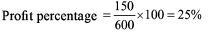QUESTION: 2

### The profit obtained by selling a book for Rs 56 is the same as the loss obtained by selling this book for Rs 42. What is the cost price of the book?

Solution:

Let us assume that the cost price = Rs X

56 - x = x - 42

2x = 98

Hence, x = 49

QUESTION: 3

### By selling a CD for Rs 150, a shop owner lost 1/16 of what it costs. What is the cost price of CD?

Solution: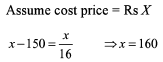QUESTION: 4

A man sold an article at a profit of 10%. If he had charged Rs 45 more his profit percentage would have been 25%. What is the cost price?

Solution: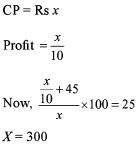QUESTION: 5

A man buys 200 oranges for Rs 10. How many oranges a rupee can he sell so that his profit percentage is 25%?

Solution: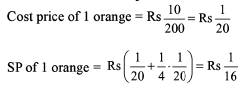So, he should sell 16 oranges in a rupee to make a profit of 25%.

QUESTION: 6

By selling an article for Rs 360, the loss incurred is 10%. At what minimum price should he sell that article to avoid loss?

Solution: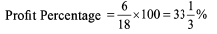QUESTION: 7

The owner of Book Point does not get either profit or loss by selling 15 books for Rs 225. How many books should he sell for the same amount to gain 25% profit?

Solution:

CP of 1 book = Rs 15 SP of 1 book in order to gain 25% profit = Rs 18.75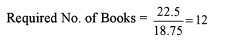QUESTION: 8

CP of 12 apples is equal to the SP of 9 apples and the discount on 10 apples is equal to the profit on 5 apples. What is the percentage difference between the CP and SP of apples?

Solution: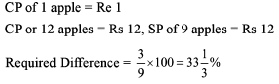QUESTION: 9

A shopkeeper professes to sell his articles at CP but gives only N grams in the place of 1000 gm. If his profit percentage is 100%, what is the value of N?

Solution:

B
(error÷1000-error)×100=1
0
(X/1000-x)×100= 100
100x = 100×(1000-x)

100x = 100000-100x

200x = 100000

X = 100000/200

X = 500

so error is 500 then N= 1000-500=500

QUESTION: 10

A car is sold for Rs 2,400 at a profit of 20%. What is the CP?

Solution: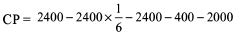QUESTION: 11

A car is sold for Rs 2,400 at a profit of 20% over SR What is the actual profit percentage?

Solution: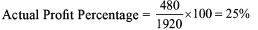QUESTION: 12

Sharat sells some articles to Chandra at a profit of 20%. Chandra now sells this article to Mayank at a loss of 30% and Mayank sells this article at a profit of 20%. If CP of Chandra is Rs 150, then what is the SP of Mayank?

Solution:

CP of Mayank = CP of Chandra * 0.7 = Rs 150 x 0.7 = Rs 105 SP of Mayank = Rs 126

QUESTION: 13

A shopkeeper wants to earn a profit of 20% and at the same time, the minimum discount which he wants to offer is 25%. What should be the minimum percentage mark-up over CP?

Solution:

Let CP = Rs 100

SP = Rs 120

MP = x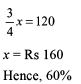QUESTION: 14

An article when sold at 10% discount on the marked price gave a profit of Rs 70. What is the CP?

Solution:

Without knowing the profit percentage, we cannot determine the CR

QUESTION: 15

A milkman buys two cows for Rs 750. He sells first cow at a profit of 22% and the second cow at a loss of 8%. What is the SP of second cow if in the whole transaction there is no profit no loss?

Solution: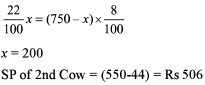Use Code STAYHOME200 and get INR 200 additional OFF Use Coupon Code